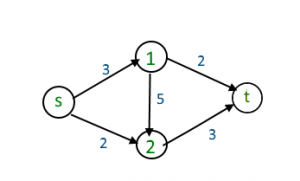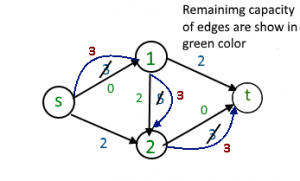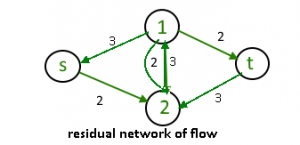# Max Flow Problem Introduction

• Difficulty Level : Medium
• Last Updated : 01 Apr, 2019

Maximum flow problems involve finding a feasible flow through a single-source, single-sink flow network that is maximum.
Let’s take an image to explain how the above definition wants to say.Each edge is labeled with capacity, the maximum amount of stuff that it can carry. The goal is to figure out how much stuff can be pushed from the vertex s(source) to the vertex t(sink).

.maximum flow possible is : 23

Following are different approaches to solve the problem :

1. Naive Greedy Algorithm Approach (May not produce an optimal or correct result)
Greedy approach to the maximum flow problem is to start with the all-zero flow and greedily produce flows with ever-higher value. The natural way to proceed from one to the next is to send more flow on some path from s to t
How Greedy approach work to find the maximum flow :

```E number of edge
f(e) flow of edge
C(e) capacity of edge

1) Initialize : max_flow = 0
f(e) = 0 for every edge 'e' in E

2) Repeat search for an s-t path P while it exists.
a) Find if there is a path from s to t using BFS
or DFS. A path exists if f(e) < C(e) for
every edge e on the path.
b) If no path found, return max_flow.
c) Else find minimum edge value for path P

// Our flow is limited by least remaining
// capacity edge on path P.
(i) flow = min(C(e)- f(e)) for path P ]
max_flow += flow
(ii) For all edge e of path increment flow
f(e) += flow

3) Return max_flow
```

Note that the path search just needs to determine whether or not there is an s-t path in the subgraph of edges e with f(e) < C(e). This is easily done in linear time using BFS or DFS.There is a path from source (s) to sink(t) [ s -> 1 -> 2 -> t] with maximum flow 3 unit ( path show in blue color )After removing all useless edge from graph it’s look likeFor above graph there is no path from source to sink so maximum flow : 3 unit But maximum flow is 5 unit. to over come form this issue we use residual Graph.

2. Residual Graphs

The idea is to extend the naive greedy algorithm by allowing “undo” operations. For example, from the point where this algorithm gets stuck in above image, we’d like to route two more units of flow along the edge (s, 2), then backward along the edge (1, 2), undoing 2 of the 3 units we routed the previous iteration, and finally along the edge (1,t)backward edge : ( f(e) ) and forward edge : ( C(e) – f(e) )

We need a way of formally specifying the allowable “undo” operations. This motivates the following simple but important definition, of a residual network. The idea is that, given a graph G and a flow f in it, we form a new flow network Gf that has the same vertex set of G and that has two edges for each edge of G. An edge e = (1,2) of G that carries flow f(e) and has capacity C(e) (for above image ) spawns a “forward edge” of Gf with capacity C(e)-f(e) (the room remaining) and a “backward edge” (2,1) of Gf with capacity f(e) (the amount of previously routed flow that can be undone). source(s)- sink(t) paths with f(e) < C(e) for all edges, as searched for by the naive greedy algorithm, corresponding to the special case of s-t paths of Gf that comprise only forward edges.

The idea of residual graph is used The Ford-Fulkerson and Dinic’s algorithms

This article is contributed by Nishant Singh . If you like GeeksforGeeks and would like to contribute, you can also write an article using contribute.geeksforgeeks.org or mail your article to contribute@geeksforgeeks.org. See your article appearing on the GeeksforGeeks main page and help other Geeks.